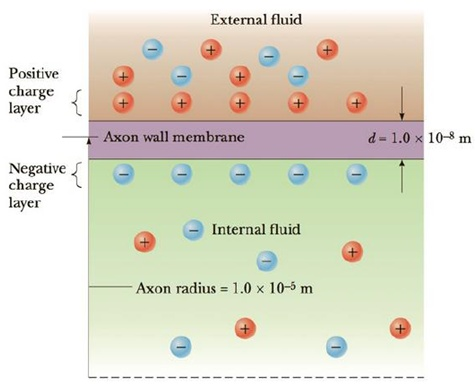Chapter 18, Problem 43P

Chapter
Section
Textbook Problem

Assume a length of axon membrane of about 0.10 m is excited by an action potential (length excited = nerve speed × pulse duration = 50.0 m/s × 2.0 × 10−3 s = 0.10 m). In the resting state, the outer surface of the axon wall is charged positively with K+ ions and the inner wall has an equal and opposite charge of negative organic ions, as shown in Figure P18.43. Model the axon as a parallel-plate capacitor and take C = κϵ0A/d and Q = C ΔV to investigate the charge as follows. Use typical values for a cylindrical axon of cell wall thickness d = 1.0 × 10−8 m, axon radius r = 1.0 × 101 μm, and cell-wall dielectric constant κ = 3.0. (a) Calculate the positive charge on the outside of a 0.10-m piece of axon when it is not conducting an electric pulse. How many K+ ions are on the outside of the axon assuming an initial potential difference of 7.0 × 10−2 V? Is this a large charge per unit area? Hint: Calculate the charge per unit area in terms of electronic charge e per squared (Å2). An atom has a cross section of about 1 Å2 (1 Å = 10−10 m). (b) How much positive charge must flow through the cell membrane to reach the excited state of + 3.0 × 10−2 V from the resting state of −7.0 × 10−2 V? How many sodium ions (Na+) is this? (c) If it takes 2.0 ms for the Na+ ions to enter the axon, what is the average current in the axon wall in this process? (d) How much energy does it take to raise the potential of the inner axon wall to + 3.0 × 10−2 V, starting from the resting potential of −7.0 × 10−2 V?Figure P18.43 Problem 43 and 44.

(a)

To determine
The positive charge on the outside of axon piece and number of K+ ions.

Explanation

Given info: The length of the axon membrane (l) is 0.10 m. Thickness of the cell wall is d=1.0×108m . Radius of the axon is r=1.0×101μm . The cell wall dielectric constant is κ=3 . The initial potential difference is 7.0×102V .

Explanation:

Formula to calculate the charge is,

Q=C(ΔV)

• C is the capacitance.
• ΔV is the potential difference.

Formula to calculate the capacitance is,

• ε0 is the permittivity of free space.
• A is the cross sectional area.

The area A is given by,

A=2πrl

From the above equations,

Q=(κ2πε0rld)(ΔV)

Substitute 8.85×1012m3kg1s4A2 for ε0 , 1.0×101μm for r, 3 for κ , 0.10 m for l and 7.0×102V for ΔV .

Q=(3)(8.85×1012m3kg1s4A2)(2π1.0×108m)(1

(b)

To determine
The positive charge on the outside of axon piece and number of Na+ ions at the excited state.

(c)

To determine
The average current in the axon wall.

(d)

To determine
The energy required.

Still sussing out bartleby?

Check out a sample textbook solution.

See a sample solution

The Solution to Your Study Problems

Bartleby provides explanations to thousands of textbook problems written by our experts, many with advanced degrees!

Get Started